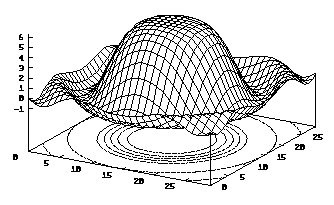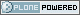# ICTP Portal

##### Sections
« September 2020 »
Su Mo Tu We Th Fr Sa
1 2 3 4 5
6 7 8 9 10 11 12
13 14 15 16 17 18 19
20 21 22 23 24 25 26
27 28 29 30

You are here: Home Gnuplot and Gnufit

# Gnuplot and Gnufit

## GnuPlot

This is a brief introduction to GnuPlot. There is also the original user's manual available on-line.

To start `gnuplot` in UNIX type

```              gnuplot
```

and the prompt `gnuplot>` will appear on the screen.

Gnuplot has a hierarchical help system. It displays the requested information screen-wise (press Enter to get to the next page) and then usually displays a list of sub-items. You can then enter one of them to get information about it, or just press Enter to go back one level (or leave the help system).

You enter the help system by typing the command `help`, optionally followed by a command name, if you already know what you're looking for. Having worked through the very basic examples presented here, you should take a closer look at the many options Gnuplot offers.

### Plotting 2-D functions

You can plot a function using the `plot` command:

```  plot sin(x)
```

To specify the plot range, write:

```  plot [x=-10:10] sin(x)
```

To overlay two function plots:

```  plot [x=-10:10] sin(x),cos(x)
```

### Plotting 3-D functions

Use the `splot` command:

```   set contour
set hidden3d
splot [-pi:pi] [-pi:pi] sin(x*x+y*y)/(x*x+y*y)
```To alter the view point type, for example

```   set view 75, 60, 1, 1
```

It rotates about the screen horizontal axis by 75 degrees, about the screen vertical by 60 degrees, and scales the x and y directions by unity.

To strip off the labeling detail use, e.g.,

```   set noborder
set nokey
set nolabel
set noxzeroaxis
set noyzeroaxis
set noxtics
set noyticks
set nozticks
```

### Plotting Data

You can also plot numerical data saved in a text file. Gnuplot expects one data point per line. Lines that start with the hash symbol (#) are ignored. Empty lines mark the end of one graph and the beginning of the next.

For instance, let's assume that you have a data file called xy.dat looking like:

```   # my experimental data
#  X     Y
1.0   1.3
2.1   4.2
3.0   9.5
```

To plot the data, write:

```   plot 'xy.dat' with lines
```

If you omit `with lines`, Gnuplot will not connect the data points.

Three dimensional data on the other hand must be saved in a different form:

```   x1
y1
z1

x2
y2
z2

x3
y3
z3```

Use the commands

```        set noparametric
set contour
set hidden3d
splot "datafile.dat" with lines
```

To have a contour plot, write

```        set contour
set nosurface
set view 0, 0, 1, 1
```

### Useful options

```  set title "plot of ..."      places a title above the plot box
set nokey                    removes the plot key (6)
set xlabel "argument ..."
set ylabel "function ..."    ylabel titles rotates to
vertical under postscript
set size  0.5, 0.5          for scaling the x and y directions
set logscale x               to set Log scaling
set samples  1000        More resolution (beyond the
default of 160) is gained with.(7)```

When you begin to use a lot of options, it is a good idea to save the commands in a text file and load them from there with the `load` command. This saves you the trouble of re-entering everything when you want to make small modifications to the output.

If Gnuplot doesn't offer you all the features you are looking for, try Mathematica (see section Mathematica).

### Printing and exporting the output

If you want to print the output, create a PostScript file first:

```   set terminal postscript
set output "output.ps"
replot
```

From outside Gnuplot (either quit or open another terminal window), send the file to the printer:

`   lpr -Pprintername output.ps`

If you want to include the output in a LaTeX document, create an EPS file instead:

```   set terminal postscript eps
set output "figure.eps"
replot
```

See section Including figures in Latex on how to proceed.

### Curve Fitting

GNUFIT is a nonlinear least squares fit mechanism into `gnuplot`.

It incorporates a nonlinear least squares fit mechanism into `gnuplot`. The fit uses the Marquardt-Levenberg-algorithm for fitting and the `gnuplot` function evaluation mechanism for calculation.

It may fit every user-defined function to any set of data pairs (x,y). Note that (x,y) and the function's return type MUST be real!

Any variable occuring in the function body may serve as fit parameter (fitting functions without adjustable parameters make no sense).

### Using GNUFIT

You must have

• a text file with data points as could be used for 2D plotting (see section Plotting Data),
• a function in x with parameters that will be modified in order to make the function fit the data, e.g.
```  f(x) = exp(a1*x) * (a2*cos(a3*x) + a4*sin(a2*x))
```
• a text file that contains the initial values for the paramters:
```  a1 = 1
a2 = 1
a3 = 1
a4 = 1
```

The syntax would then be

```   fit f(x) 'datafile' via 'parameter file'
```

Special `gnuplot` variables:

• FIT_INDEX: This variable always contains the current data point number during execution starting with 1. You may use it in your fit function to implement multiple-branch-fits.
• FIT_SKIP: You may specify a positive integer to always skip i data points during fitting. This increases execution speed by the price of less exact results.
• FIT_LIMIT: may be specified to change the default epsilon limit (1e-5). When the sum of squared residuals changes between 2 iteration steps by less than a factor of this number, the fit is considered as 'converged'.

### Example

```    FIT_LIMIT = 1e-10
FIT_SKIP = 2
f(x)=exp(a1*x)*( a2*cos(a3*x) + a4*sin(a2*x) )
fit f(x) 'Jo(x)-data' 'guess.a'
#
g(x)=b1+b2*x+b3*x**2+b4*x**3+b5*x**4+b6*x**5
fit g(x) 'Jo(x)-data' 'guess.b'
#
plot f(x), g(x), 'Jo(x)-data'
```

would result inIn this example, two different analytical functions (`f(x)` and `g(x)`) have been used to fit the bessel function data set in the file "Jo(x)-data" by starting the fitting with the guess parameter files "guess.a" for the parameters `a1`, ..., `a4` and "guess.b" for `b1`,..., `b6`.

The file "guess.a" should look like this:

```             a1 = 1
a2 = 1
a3 = 1
a4 = 1
```

### `update` parameters

After the plot appeared in your graphics terminal (as in the figure above), you may `update` the input file 'guess.a' (containing the initial guess `a1`, `a2`, etc) by simply typing

```   update "filename"
```

This command updates the start parameter assignments in a start parameter file (in the previous example this is guess.a). Each parameter will be replaced by its actual value. This is useful for restarting a converged or stopped fit again.

For example the `update` "guess.a" should now look like this

```            a1              = -0.0826758
a2              = 0.800099
a3              = 0.955014
a4              = 0.499098
```

Then repeat again the gnufit procedure for obtaining a new improved fit of your data set (by simply loading the file containing the `gnuplot` command as in the example of the previous subsubsection).

### `fit.log` file

After each iteration step a detailed info is given about the fit's state both on the screen and on a so-called logfile

```   fit.log
```

This file will never be erased but always appended so that the fit's history isn't lost.This site conforms to the following standards: